Back to Kemp Acoustics HomeNext: Issues in multimodal bore Up: Multimodal reflections Previous: Multimodal reflectance of a   Contents

# Multimodal input impulse response

Now we will use an inverse Fourier transform of the plane reflectance to give the plane component of the input impulse response of our simple discontinuity between cylindrical pipes. A similar calculation was performed by Boone et al.  for the input impulse response of a lightly damped rectangular cavity. In order to work out the inverse Fourier transform we need to calculate the reflectance for a number of equally spaced frequencies. The zero frequency component cannot be worked out with the present multimodal method because
means that
has elements which go to infinity. It may, however, be noted that the plane reflectance tends to the plane wave approximation reflection coefficient at low frequency, so theplane reflectance is taken from equation (2.20) in what follows.

When the Fourier transform of a real signal is performed the result is a conjugate symmetric spectrum. That is, the real part of the spectrum is symmetric and the imaginary part anti-symmetric. The point of symmetry is called the Nyquist frequency, and has a value half that of the sample frequency. We require that there are no significant components in the signal above the Nyquist frequency to prevent numerical errors due to aliasing. Similarly, when we calculate an inverse Fourier transform, we require that the spectrum decays to zero by the Nyquist frequency. From figure 6.2 we note that if we go to very high frequencies the plane reflectance does indeed decay to zero, meaning that numerical problems may be avoided if the Nyquist frequency chosen is high enough. The vector of the plane reflectance must then be made conjugate symmetric for the inverse Fourier transform to be calculated .

The result of an inverse Fourier transform of the plane reflectance is shown in figure 6.5 for a radius ratio of. Progressively higher values for the Nyquist frequency in the calculation of the plane reflectance were taken until the inverse transform showed convergence on a final answer. The appearance of the time response is that of a negative pulse. This is to be expected as we are calculating the reflection of an impulse from a expansion which has a negative reflection coefficient. The finite width of the pulse shows how the high frequency components have been removed and the oscillations in the time domain response correspond to the peak in the plane reflectance due to the lowest frequency cut-off.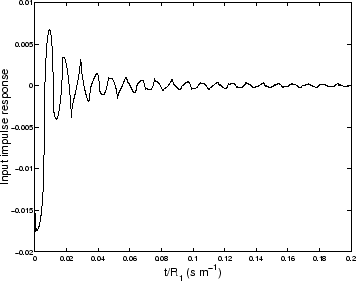The results presented so far are for scattering from a junction between two infinite pipes. In order to treat physically realisable systems we want to have a method of calculating the input impulse response of any musical instrument approximated by a series of cylinders. This is achievable by first calculating the input impedance matrix by the multimodal method described in section 2.6. The reflectance matrix can then be found using equation (6.8). The plane wave component of the input impulse response can be calculated by taking the inverse Fourier transform of the plane reflectance in the same manner as in section 6.4. It should be noted that this method involves calculating the multimodal radiation impedance; this can be readily done if the instrument is assumed to have an infinite baffle termination. It is logical that the input impulse response should not depend on the radiation impedance for the first
seconds taken for sound to reflect from the radiating end and arrive back at the input where
is the length of the instrument.

Back to Kemp Acoustics HomeNext: Issues in multimodal bore Up: Multimodal reflections Previous: Multimodal reflectance of a   Contents
Jonathan Kemp 2003-03-24